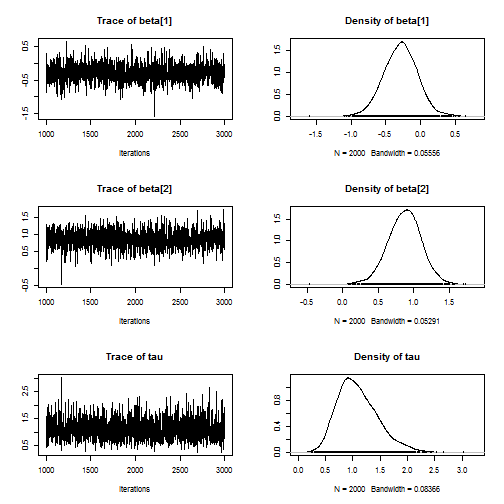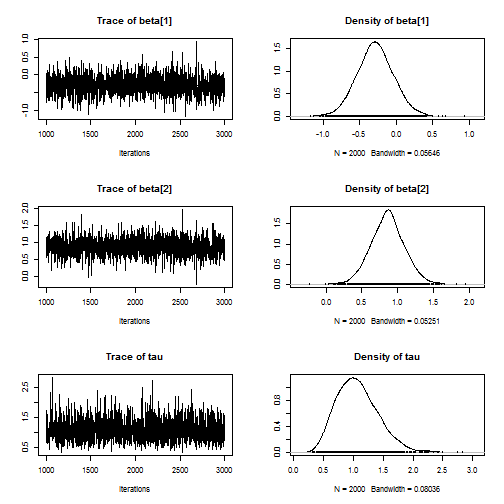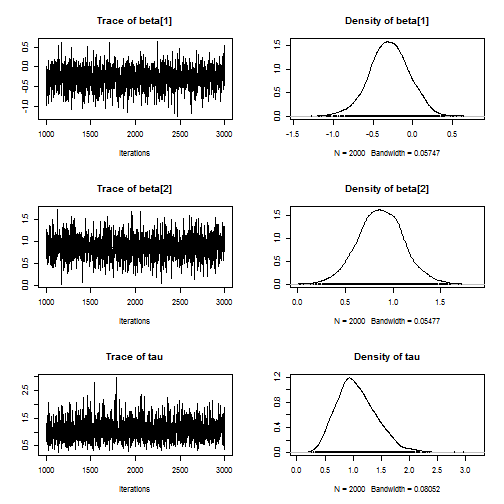# Understanding error messages in JAGS

In the following code errors are intentially injected onto JAGS runs with the goal of understanding the error messages and ultimately to be able to decipher the errors messages when real unintentional errors are made. We will work with a simulated simple linear regression model.

``````# Generate data
n <- 20
p <- 2
X <- rnorm(n)
Y <- rnorm(n,X,1)

# Hyperpriors

prec_beta <- 0.01
a         <- 0.01
b         <- 0.01
``````

## (0) Correct model

`````` model_string <- "model{

# Likelihood
for(i in 1:n){
Y[i] ~ dnorm(beta + X[i]*beta,tau)
}

# Prior
for(j in 1:p){beta[j] ~ dnorm(0, prec_beta)}
tau ~ dgamma(a,b)

}"

library(rjags)
data  <- list(Y=Y,X=X,p=p,n=n,prec_beta=prec_beta,a=a,b=b)
keep  <- c("beta","tau")

model <- jags.model(textConnection(model_string), data = data,quiet=TRUE)
update(model, 1000, progress.bar="none")
samp  <- coda.samples(model, variable.names=keep, n.iter=2000, progress.bar="none")
plot(samp)
```````````` rm(samp,model,data,keep)
``````

## (1) Forget to pass a variable as data

The error message clearly says that we forgot to pass “b” in “## Unknown variable b”. Since there is an error in this step the objects “model” and “samps” are not created and this lead to many error messages down the line.

`````` model_string <- "model{

# Likelihood
for(i in 1:n){
Y[i] ~ dnorm(beta + X[i]*beta,tau)
}

# Prior
for(j in 1:p){beta[j] ~ dnorm(0, prec_beta)}
tau ~ dgamma(a,b)

}"

library(rjags)
data  <- list(Y=Y,X=X,p=p,n=n,prec_beta=prec_beta,a=a) # Drop b!
keep  <- c("beta","tau")

model <- jags.model(textConnection(model_string), data = data,quiet=TRUE)
``````
``````## Error in jags.model(textConnection(model_string), data = data, quiet = TRUE): RUNTIME ERROR:
## Compilation error on line 10.
## Unknown variable b
## Either supply values for this variable with the data
## or define it  on the left hand side of a relation.
``````
`````` update(model, 1000, progress.bar="none")
``````
``````## Error in update(model, 1000, progress.bar = "none"): object 'model' not found
``````
`````` samp  <- coda.samples(model, variable.names=keep, n.iter=2000, progress.bar="none")
``````
``````## Error in coda.samples(model, variable.names = keep, n.iter = 2000, progress.bar = "none"): object 'model' not found
``````
`````` plot(samp)
``````
``````## Error in plot(samp): object 'samp' not found
``````
`````` rm(samp,model,data,keep)
``````
``````## Warning in rm(samp, model, data, keep): object 'samp' not found
``````
``````## Warning in rm(samp, model, data, keep): object 'model' not found
``````

## (2) Missing values

The error message clearly says “Unable to resolve the following parameters: ## X1”. It even provides solutions! It says “Either supply values for these nodes with the data or define them on the left hand side of a relation.” That is, to fix the problem you either put something in for X and resend or give X a prior.

`````` model_string <- "model{

# Likelihood
for(i in 1:n){
Y[i] ~ dnorm(beta + X[i]*beta,tau)
}

# Prior
for(j in 1:p){beta[j] ~ dnorm(0, prec_beta)}
tau ~ dgamma(a,b)

}"

library(rjags)
X_miss    <- X  # Add a missing X
X_miss <- NA

data  <- list(Y=Y,X=X_miss,p=p,n=n,prec_beta=prec_beta,a=a,b=b)
keep  <- c("beta","tau")

model <- jags.model(textConnection(model_string), data = data,quiet=TRUE)
``````
``````## Error in jags.model(textConnection(model_string), data = data, quiet = TRUE): RUNTIME ERROR:
## Unable to resolve the following parameters:
## X (line 5)
## Either supply values for these nodes with the data
## or define them on the left hand side of a relation.
``````
`````` update(model, 1000, progress.bar="none")
``````
``````## Error in update(model, 1000, progress.bar = "none"): object 'model' not found
``````
`````` samp  <- coda.samples(model, variable.names=keep, n.iter=2000, progress.bar="none")
``````
``````## Error in coda.samples(model, variable.names = keep, n.iter = 2000, progress.bar = "none"): object 'model' not found
``````
`````` plot(samp)
``````
``````## Error in plot(samp): object 'samp' not found
``````
`````` rm(samp,model,data,keep,X_miss)
``````
``````## Warning in rm(samp, model, data, keep, X_miss): object 'samp' not found
``````
``````## Warning in rm(samp, model, data, keep, X_miss): object 'model' not found
``````

## (3) Defining a variables twice

Here Y's likelhood is defined n times because I changed “Y[i]” to “Y” inside the loop. It complains that “## Attempt to redefine node Y”.

`````` model_string <- "model{

# Likelihood
for(i in 1:n){
Y ~ dnorm(beta + X[i]*beta,tau)  # It should be Y[i] not Y
}

# Prior
for(j in 1:p){beta[j] ~ dnorm(0, prec_beta)}
tau ~ dgamma(a,b)

}"

library(rjags)
data  <- list(Y=Y,X=X,p=p,n=n,prec_beta=prec_beta,a=a,b=b)
keep  <- c("beta","tau")

model <- jags.model(textConnection(model_string), data = data,quiet=TRUE)
``````
``````## Error in jags.model(textConnection(model_string), data = data, quiet = TRUE): RUNTIME ERROR:
## Compilation error on line 5.
## Attempt to redefine node Y
``````
`````` update(model, 1000, progress.bar="none")
``````
``````## Error in update(model, 1000, progress.bar = "none"): object 'model' not found
``````
`````` samp  <- coda.samples(model, variable.names=keep, n.iter=2000, progress.bar="none")
``````
``````## Error in coda.samples(model, variable.names = keep, n.iter = 2000, progress.bar = "none"): object 'model' not found
``````
`````` plot(samp)
``````
``````## Error in plot(samp): object 'samp' not found
``````
`````` rm(samp,model,data,keep)
``````
``````## Warning in rm(samp, model, data, keep): object 'samp' not found
``````
``````## Warning in rm(samp, model, data, keep): object 'model' not found
``````

## (4) Priors with invalid range or intial values

Here the prior for the variance is negative. The same error often occurs when the initial values of not legitimate. It says

“ Error in node Y ## Invalid parent values”

This is confusing. I take this to mean that Y's parent nodes are its mean and precision, and that the precision is negative and thus invalid.

`````` model_string <- "model{

# Likelihood
for(i in 1:n){
Y[i] ~ dnorm(beta + X[i]*beta,tau)  # It should be Y[i] not Y
}

# Prior
for(j in 1:p){beta[j] ~ dnorm(0, prec_beta)}
tau ~ dunif(-2,-1) # Crazy prior!

}"

library(rjags)
data  <- list(Y=Y,X=X,p=p,n=n,prec_beta=prec_beta)
keep  <- c("beta","tau")

model <- jags.model(textConnection(model_string), data = data,quiet=TRUE)
``````
``````## Error in jags.model(textConnection(model_string), data = data, quiet = TRUE): Error in node Y
## Invalid parent values
``````
`````` update(model, 1000, progress.bar="none")
``````
``````## Error in update(model, 1000, progress.bar = "none"): object 'model' not found
``````
`````` samp  <- coda.samples(model, variable.names=keep, n.iter=2000, progress.bar="none")
``````
``````## Error in coda.samples(model, variable.names = keep, n.iter = 2000, progress.bar = "none"): object 'model' not found
``````
`````` plot(samp)
``````
``````## Error in plot(samp): object 'samp' not found
``````
`````` rm(samp,model,data,keep)
``````
``````## Warning in rm(samp, model, data, keep): object 'samp' not found
``````
``````## Warning in rm(samp, model, data, keep): object 'model' not found
``````

## (5) Passing variables that are not used

Here “a” and “b” are not used in the JAGS model but still passed to JAGS, giving the warning “Unused variable "a” in data". In this case it still runs, but it would better to remove a and b from the data list.

`````` model_string <- "model{

# Likelihood
for(i in 1:n){
Y[i] ~ dnorm(beta + X[i]*beta,tau)
}

# Prior
for(j in 1:p){beta[j] ~ dnorm(0, prec_beta)}
tau ~ dgamma(.1,.1) # No a or b!

}"

library(rjags)
data  <- list(Y=Y,X=X,p=p,n=n,prec_beta=prec_beta,a=a,b=b)
keep  <- c("beta","tau")

model <- jags.model(textConnection(model_string), data = data,quiet=TRUE)
``````
``````## Warning in jags.model(textConnection(model_string), data = data, quiet =
## TRUE): Unused variable "a" in data
``````
``````## Warning in jags.model(textConnection(model_string), data = data, quiet =
## TRUE): Unused variable "b" in data
``````
`````` update(model, 1000, progress.bar="none")
samp  <- coda.samples(model, variable.names=keep, n.iter=2000, progress.bar="none")
plot(samp)
```````````` rm(samp,model,data,keep)
``````

## (6) Sending an invalid list of parameters to keep

Here theta is in the variable list but not the model and it complains that it “Failed to set trace monitor for theta ## Variable theta not found”. In this case it still runs, but it would better to remove theta from the parameter list.

`````` model_string <- "model{

# Likelihood
for(i in 1:n){
Y[i] ~ dnorm(beta + X[i]*beta,tau)
}

# Prior
for(j in 1:p){beta[j] ~ dnorm(0, prec_beta)}
tau ~ dgamma(a,b)

}"

library(rjags)
data  <- list(Y=Y,X=X,p=p,n=n,prec_beta=prec_beta,a=a,b=b)
keep  <- c("beta","tau","theta") # Ah!

model <- jags.model(textConnection(model_string), data = data,quiet=TRUE)
update(model, 1000, progress.bar="none")
samp  <- coda.samples(model, variable.names=keep, n.iter=2000, progress.bar="none")
``````
``````## Warning in jags.samples(model, variable.names, n.iter, thin, type = "trace", : Failed to set trace monitor for theta
## Variable theta not found
``````
`````` plot(samp)
```````````` rm(samp,model,data,keep)
``````